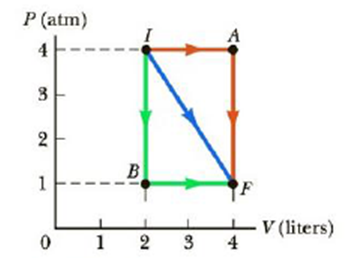Chapter 12, Problem 5P

Chapter
Section
Textbook Problem

A gas expands from I to F along the three paths indicated in Figure P12.5. Calculate the work done on the gas along paths (a) IAF, (b) IF, and (c) IBF.Figure P12.5 Problems 5 and 15.

(a)

To determine
The work done along the path IAF.

Explanation

Section1:

To determine: The work done along the path IA.

Answer: Thus, the work done is 810J .

Explanation:

Given Info:

Constant pressure of the gas is (4atm)(1.013×105Pa/atm) .

Initial volume ( Vi ) of the gas is 2L .

Finial volume ( Vf ) of the gas is 4L .

Formula to calculate the work done of the gas is,

W=P(VfVi)

• P is the pressure
• Vf is the final volume
• Vi is the initial volume

Substitute 4atm for P , 4L for Vf and 2L for Vi to find the work done,

W=[(4atm)(1.013×105Pa/atm)](4L2L)[103m31L]=810J

Thus, the work done is 810J

(b)

To determine
The work done along the path IF.

(c)

To determine
The work done along the path IBF.

Still sussing out bartleby?

Check out a sample textbook solution.

See a sample solution

The Solution to Your Study Problems

Bartleby provides explanations to thousands of textbook problems written by our experts, many with advanced degrees!

Get Started

Find more solutions based on key concepts